## ML Aggarwal Class 9 Solutions for ICSE Maths Chapter 6 Problems on Simultaneous Linear Equations Chapter Test

Question 1.
A 700 gm dry fruit packcosts Rs. 216. It contains some almonds and the rest cashew kernel. If almonds cost Rs. 288 ‘per kg and cashew kernel cost Rs. 336 per kg, what are the quantities of the two dry fruits separately ?
Cost of 700 gm of fruit = Rs. 216
Cost of almonds = Rs. 288 per kg
and cost of cashew = Rs. 336 per kg
Let weight of almond = x gm
Then weigh of cashew = (700 – x) gm
According to the sum,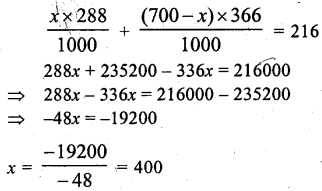∴ weight of almond = 400 gm
and weight of cashew = 700 – 400
= 300 gm

Question 2.
Drawing pencils cost 80 paise each and coloured pencils cost Rs. 1.10 each. If altogether two dozen pencils cost Rs. 21.60, how many coloured pencils are there ?
Let the number of drawing pencils = x
and let the number of coloured pencils = y
Then, according to first condition of given problem,
x + y = 2 × 12 (2 dozen = 2 × 12)
⇒ x + y = 24 ….(1)
According to second condition of given problem,Substituting the value ofy in equation (1), we get
x + 8 = 24 ⇒ x = 24 – 8 ⇒ x = 16
Hence, Number of coloured pencils y = 8

Question 3.
Shikha works in a factory. In one week she earned Rs. 390 for working 47 hours, of which 7 hours were overtime. The next week she earned Rs. 416 for working 50 hours, of which 8 hours were overtime. What is Shikha’s hourly earning rate?
Let Shikha’s earning be Rs. x per regular hour and Rs. y per hour overtime,
then according to first condition of given problem, 40x + 7y = 390 ….(1)
According to second condition of given problem, 42x + 8y = 416 ….(2)
Multiplying equation (1) by 8 and equation (2) by 7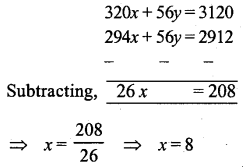Substituting the value of x in equation (1), we get
40 × 8 + 7y = 390 ⇒ 320 + 7y = 390
⇒ 7y = 390 – 320 ⇒ 7y = 70
⇒ y = 10
Hence, Shika’s earning be Rs. 8 per regular hours and Rs. 10 per hour overtime.

Question 4.
The sum of the digits of a two digit number is 7. If the digits are reversed, the new number increased by 3 equals 4 times the original number. Find the number.
Let the digit at tens place = x
and let the digit at unit place = y
then number = 10 × x + 1 × y = 10x +y
Reversing the number =10 × y + x + 1 = 10y + x
According to first condition of given problem
x + y = 7 ….(1)
According to second condition of given problem,
10y + x = 4(10x + y) – 3 ⇒ 10y + x = 40x + 4y – 3
⇒ 10y + x – 40x – 4y = -3 ⇒ -39x + 6y = -3
⇒ +3(-13x + 2y) = -3 ⇒ -3 (13 – 2y) = -3
⇒ 13x – 2y = $$\frac{-3}{-3}$$ ⇒ 13x – 2y = 1 ….(2)
Multiplying equation (1) by 2, we get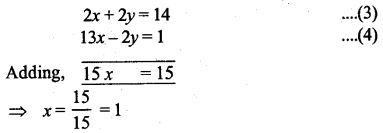Substituting the value of x in equation (1), we get
1 + y = 7 ⇒ y = 7 – 1 ⇒ y = 6
Hence, Number = 10x + y = 10 × 1 + 6
= 10 + 6 = 16

Question 5.
Three years hence a man’s age will be three times his son’s age and 7 years ago he was seven times as old as his son. How old are they now ?
Let the man’s age now = x years
and his son’s age now = y years
three years hence man’s age = (x + 3) years
three years hence his son’s age = (y + 3) years
7 years ago man’s age = (x – 7) years
7 years ago his son’s age = (y – 7) years
According to first condition of given problem,
(x + 3) = 3 (y + 3) ⇒ x + 3 – 3y + 9
⇒ x – 3y = 9 – 3 ⇒ x – 3y = 6 ….(1)
According to second condition of given problem,
(x – 7) = 7 (y – 7) ⇒ x – 7 = 7y – 49
⇒ x – 7y = -49 + 7 ⇒ x – 7y = -42 ….(2)
From equation (1) and equation (2), we getSubstituting the value ofy in equation (1), we get
x – 3 × 12 = 6 ⇒ x – 36 = 6 ⇒ x = 6 + 36
⇒ x = 42
Hence, Man’s age = 42 years
His son’s age y years = 12 years

Question 6.
Rectangles are drawn on line segments of fixed lengths. When the breadths are 6 m and 5 m respectively the sum of the areas of the rectangles is 83 m2. But if the breadths are 5 m and 4 m respectively the sum of the areas is 68 m2. Find the sum of the areas of the squares drawn on the line segments.
Let the length of first fixed line segment =x
and length of second line segment = y
then in first case sum of areas = 83 m2
and breadths are 6 m and 5 m respectively.
∴ 6x + 5y = 83 …(i)
In second case,
5x + 4y = 68 …(ii)
Multiply (i) by 4 and (ii) by 5,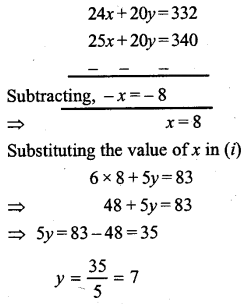Hence first line segment = 8 m
and second line segment = 7 m
Now sum of areas of the square on these two line segments = (8)2 + (7)2
= 64 + 49 = 113 m2

Question 7.
If the length and the breadth of a room are increased by 1 metre each, the area is increased by 21 square metres. If the length is decreased by 1 metre and the breadth is increased by 2 metres, the area is increased by 14 square metres. Find the perimeter of the room.
Let the length of room = x metre
and let the breadth of the room = y metres
Area of room = length × breadth = x × y sq. metre = xy sq. metre.
when the length is increased by 1 metre, then new length = (x + 1) m
when the breadth is increased by 1 metre, then new breadth = (y + 1) m
New Area = new length × new breadth
= (x + 1) (y + 1) sq. metre
when the length is decreased by 1 metre, then new length = (x – 1) m
when the breadth is increased by 2 metre, new breadth = (y + 2) m
New Area = new length × New breadth
= (x – 1) (y + 2) sq. metre
According to first condition of given problem,
xy = (x + 1)(y + 1) – 21
⇒ xy = x(y + 1) + 1 (y + 1) – 21
⇒ xy = xy + x + y + 1 – 21 ⇒ 0 = x + y – 20
⇒ 20 = x + y ⇒ x + y = 20 …(1)
According to second condition of given problem,
xy = (x – 1)(y + 2) – 14
⇒ xy = x(y + 2) – 1(y + 2) – 14
⇒ xy = xy + 2 – y – 2 – 14
⇒ 0 = 2x – y – 2 – 14 ⇒ 0 = 2x – y – 16
⇒ 16 = 2x – y ⇒ 2x – y = 16 …(2)
From equation (1) and (2), we get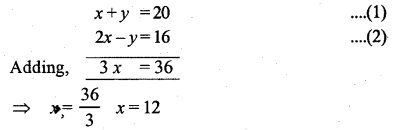Substituting the value of x in equation (1), we get
12 + y = 20 ⇒ y = 20 – 12 ⇒ y = 8
∴ Length of the room = x m = 12m
∴ Breadth of the room = y m = 8 m
Hence perimeter of the room = 2 (length + breadth)
= 2 (12 + 8) metre = 2 × 20 metre = 40 metre

Question 8.
The lenghts (in metres) of the sides of a triangle are $$2 x+\frac{y}{2}, \frac{5 x}{3}+y+\frac{1}{2} \text { and } \frac{2}{3} x+$$$$2 y+\frac{5}{2}$$. If the triangle is equilateral, find its perimeter.
Length of the sides of an equilateral triangle are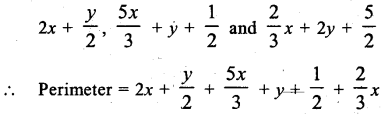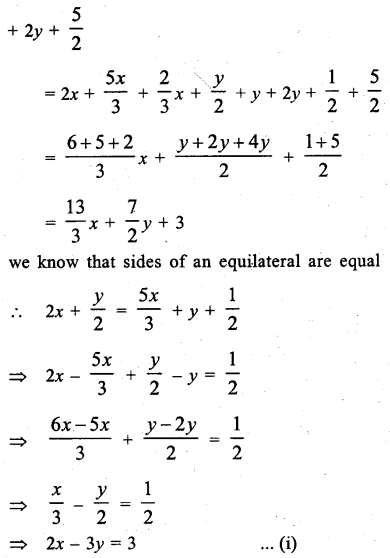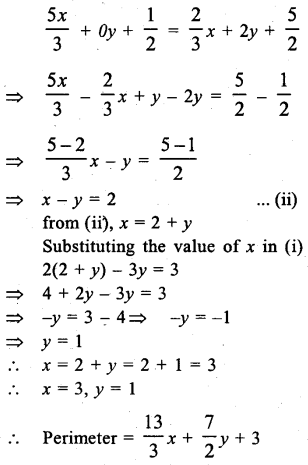Question 9.
On Diwali eve, two candles, one of which is 3 cm longer than the other are lighted. The longer one is lighted at 5.30 p.m. and the shorter at 7 p.m. At 9.30 p.m. they both are of the same length. The longer one burns out at 11.30 p.m. and the shorter one at 11 p.m. How long was each candle originally ?